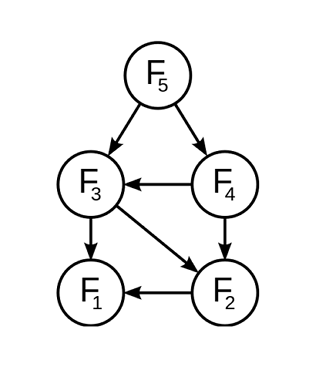Probability

# Fibonacci Numbers: Level 4 Challenges

Find the least positive integer $d$ for which there exists an infinite arithmetic progression satisfying the following properties:

1. Each term of the progression is a positive integer.
2. The common difference of the progression is $d$.
3. No term of the progression appears in the Fibonacci sequence.

Details and assumptions

The Fibonacci sequence is defined by $F_1 = 1, F_2 = 1$ and $F_{n+2} = F_{n+1} + F_{n}$ for $n \geq 1$.

The arithmetic progression has infinitely many terms.How many values of $k$ are there such that $F_k$ is of the form $2^n$?

Note that both $k$ and $n$ are non-negative integers.

Also $F_0=0,F_1=1$ and $F_m=F_{m-1}+F_{m-2}$

How many Fibonacci numbers $F_n$ divide $F_{100}$, where $n$ is a positive integer greater than 1?

Find the sum of all primes $p$, such that $p$ divides $u_p$, where $u_p$ is the $p$-th Fibonacci number.

Details and assumptions

The Fibonacci sequence is defined by $F_1 = 1, F_2 = 1$ and $F_{n+2} = F_{n+1} + F_{n}$ for $n \geq 1$.

I have a Monster, in which when I enter two positive integers $(a,b)$, where $b>a$, it gives out two positive integers, $(b,a+b)$.

I then enter these two numbers again into the Monster, and get two more numbers.

I do this process continuously $10$ times, and then add my final two numbers, to get $3935$.

What is the product of the two numbers I entered at first?

×